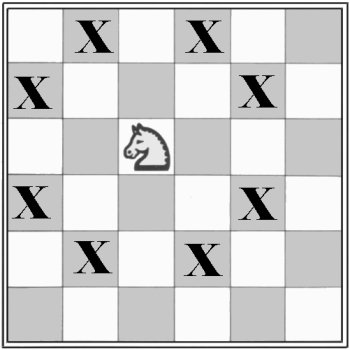A Tryst With Chess
/
No tags
Problem
Editorial
Analytics

You are given a 10X10 chessboard with a knight on coordinate (I,J). You have to find the number of blocks on the chessboard that the knight can be at in exactly N moves.

$NOTE:$

The knight can move from its positon in the diagram to all the coordinates marked by X in the following diagram in one move. For the 10X10 chessboard $(1,1)$ is the top-left corner,$(1,10)$ is the top-right corner and $(10,10)$ is the bottom-right corner.$INPUT$

Input will consist of three space seperated integers I,J,N

N is less than 10.

$OUTPUT$

Print a single integer denoting the number of blocks on the chessboard that the knight can be at in exactly N moves.

Problem Setter - Sheldon

SAMPLE INPUT
3 3 1
SAMPLE OUTPUT
8
Time Limit: 0.5 sec(s) for each input file.
Memory Limit: 256 MB
Source Limit: 1024 KB

## Contributors

Initializing Code Editor...